Select Page

Cost Volume Profit Analysis

Basically, break-even analysis determines the “break-even point”, at which operations neither make money nor lose money. At the break-even point, there is no gain or loss; hence costs or expenses are equal to revenues/incomes. Determining the break-even point for a business is the useful business planning tool.

Cost, Revenue and Profit

Following are costs and revenues are used for break-even analysis

Fixed Cost

Fixed costs are those that remain the same regardless of sales volume or production. They are expressed usually in amount or rupees say Rs. 200,000. Rent, insurance, supervisory salary and real estate taxes are usually examples of fixed cost.

• Fixed cost remains the same whether the business produces nothing or is working at full capacity
• Fixed cost per unit is variable, when production increase it decrease and vice versa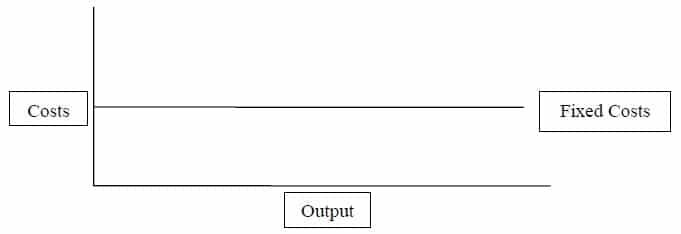Variable Cost

Variable costs are those which change as sales volume or production changes. They are expressed usually as a percent of sold units like 8% of sales. Inventory, raw materials and direct production labor, for example, are usually variable costs.

• Variable Cost =   Variable Cost per Unit x Sold Units
• Variable cost per unit is fixed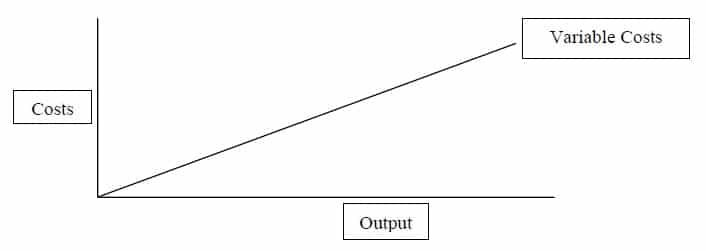Total Cost

By adding fixed and variable cost we derive total cost

Total Cost     =     Fixed Cost    +    Variable Cost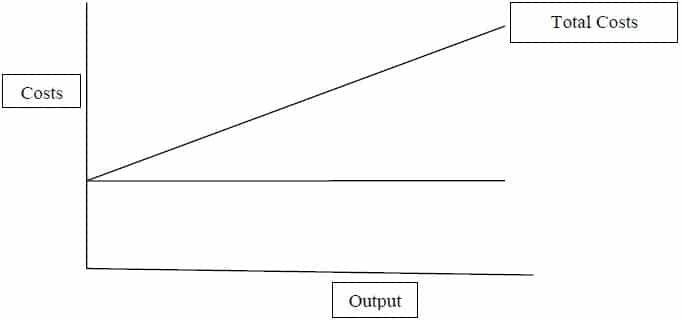Total Revenue

This is the amount of money that a firm receives from selling the product or providing services

Total Revenue     =     Price per Unit x Sold Units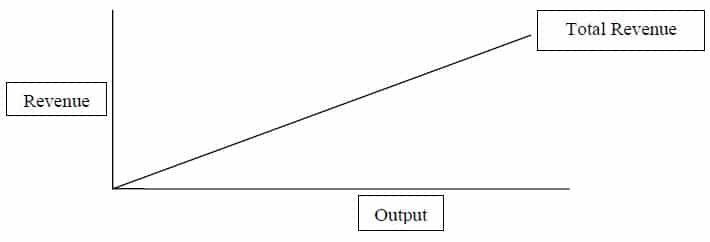Profit

Profit is net of revenue from expenses. The state when revenues are higher side and expenses are in lower side is called profit.

Profit = Total Revenue – Total Cost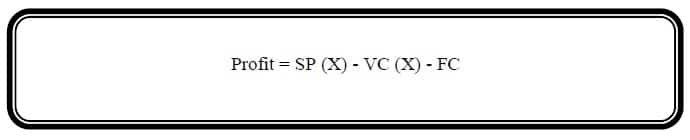SP = Selling price;                                        X = Quantity of units produced or sale

VC = Variable cost and                              FC = Fixed cost

Example # 1:

Ahmed, Inc. produces and sells a single product for Rs. 40 per unit. Costs are: variable cost per unit Rs. 30 and fixed costs Rs. 360,000. Using the profit equation determine the break-even point in units.

Solution:

Profit = SP (X) – VC (X) – FC

0 = 40 (x) – 30 (x) – 360,000

0 = 10 (x) – 360,000

360,000 = 10(x)

x =   36,000 units

>> Practice Multi-choice Questions and Answers Cost Volume Profit Analysis MCQs.

Contribution Margin

Contribution Margin is the amount of money remaining after the variable costs of producing or purchasing is subtracted from the selling price.

• Contribution margin = Selling price – Variable cost
• Contribution margin can be calculated as per unit and as a ratio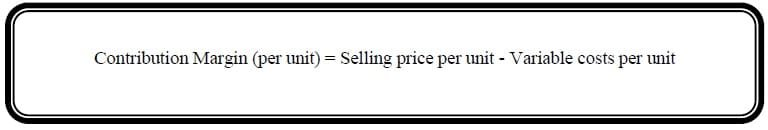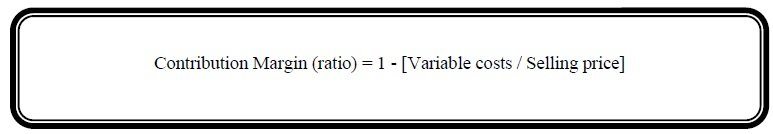>> Practice More Questions and Answers Cost Volume Profit Analysis Problems and Solutions.

Cost-Volume-Profit (CVP) Analysis

Calculating break-even is also referred to as Cost-Volume-Profit Analysis (CVP) or Contribution Analysis. Break even analysis is sometimes referred to as cost-volume-profit analysis because these are three key elements (i.e. cost, volume and profit) in the calculation. It is sometimes referred to as contribution analysis because calculating break even requires determining how many service or product contributions (selling price per unit minus variable costs per unit) are necessary to cover, or pay for, the product’s expected fixed costs. CVP Analysis includes:

1. Break-Even in units and amount
2. Margin of Safety
3. Calculating the Target Sales (in units and amount) required to achieve target profit
4. Estimated Future Profit
5. Deciding Selling Price

Break-Even in Units and Amount

Break-even is volume of sales or units at which business just ‘break even’. At break-even point total cost is equal to total revenue.  At break-even point, total contribution is just large enough to cover fixed cost. There are two methods for calculating break-even point in unit and amount:

• Mathematical equation
• Graphical presentation

Mathematical Equations for Break-even Analysis: Following are equations for Break-even analysis: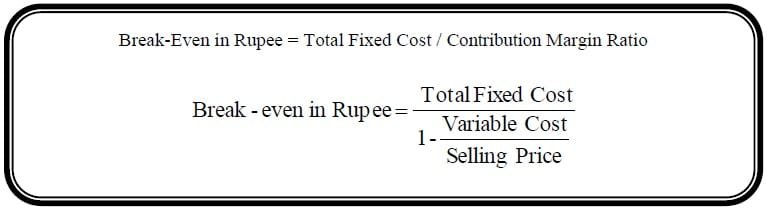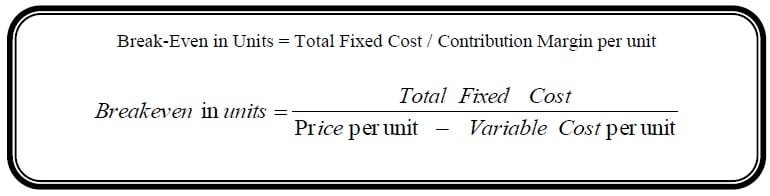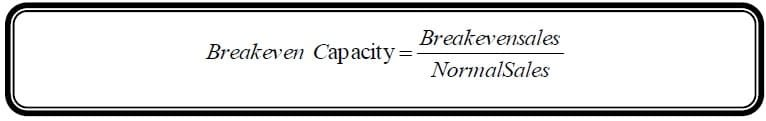Example # 2:

A Company has fixed costs of Rs. 10,000 for this period. Direct labor is Rs. 1.50 per unit, and material is Rs.  0.75 per unit. The selling price is Rs. 4.00 per unit and normal sales is Rs. 24,000. Find the break-even point in dollars and in units and also break-even capacity?

Solution:

The break-even point in dollars: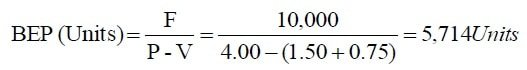The break-even point in units:The break-even point in units: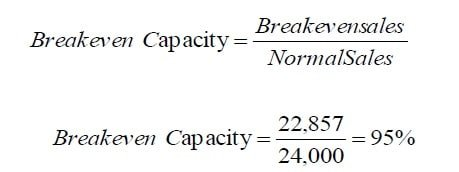>> Practice More Questions and Answers Cost Volume Profit Analysis Problems PDF.

Example # 3:

Following data is extracted from Ali (Pvt.) Ltd. You are required to calculate the break-even in rupees, in units and capacity. The data shows sales Rs. 5,000,000 and per unit sale price Rs. 4.

Accounts                       Total                            Variable                     Fixed

Material                            1,000,000                     1,000,000                         0

Labor                                 1,400,000                     1,400,000                          0

FOH                                   1,600,000                     400,000                         1,200,000

Marketing Expenses       350,000                        150,000                          200,000

Admin Expenses              250,000                       50,000                            200,000

Solution:

The break-even point in dollars: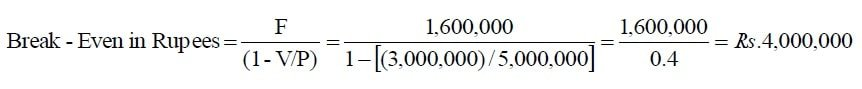The break-even point in units is: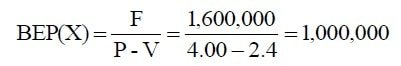The break-even capacity: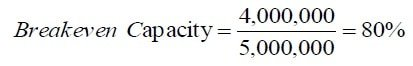>> Practice More Questions and Answers Cost Volume Profit Analysis Problems and Solutions.

Graphical Presentation for Break-even Analysis

Break-even point can be obtained by Graphical Presentation. Following steps used for graphical presentation.

1. Draw X-axis for volume of sales or units.

2. Draw Y-axis for cost and revenue.

3. A line is drawn on the graph for sales revenue. This is zero when sales unit is zero. It rises in a straight line. To draw the revenue line, you therefore need to plot one more point on the graph and join this to the origin of the graph (x = 0, y = 0).

4. Draw Fixed Cost Line. It cuts the Y-axis at the amount of fixed cost.

5. A line is then drawn for total cost. To do this, we must add variable cost to fixed cost. When sales volume is zero, variable costs are zero, so total cost = fixed cost only. To draw the total line, you therefore need to plot one more point on the graph and join this to the fixed cost at zero sales volume (x = 0 and y = fixed cost).

6. Total Cost Line intersect the Sales Line at the point of Break-Even.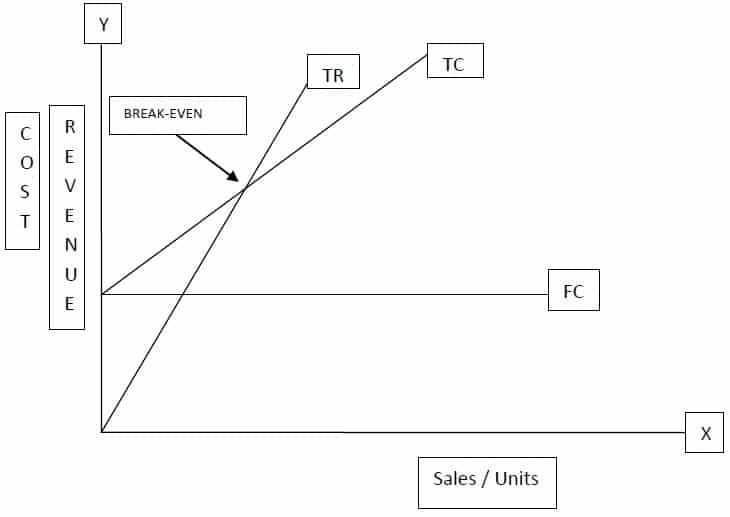>> Practice More Questions and Answers Cost Volume Profit Analysis Problems and Solutions.

>>> Practice More Questions and Answers Cost Volume Profit Analysis Problems and Solutions.

Margin of Safety

Actual sales volume may not be the same as budgeted sales volume. Actual sales may fall short or exceed to budget. The difference between the budgeted sales volume and the break-even sales volume is known as the margin of safety. It usually expressed as a percentage of budgeted sales (100/Budgeted Sales*Margin of Safety).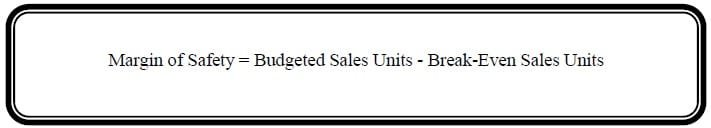Example # 4:

Following data extracted from the books of ABC Company:

Budgeted sales                     80,000 units                                          Selling price           Rs. 8

Variable cost                        Rs. 4 per unit                                        Fixed cost              Rs. 200,000

Solution: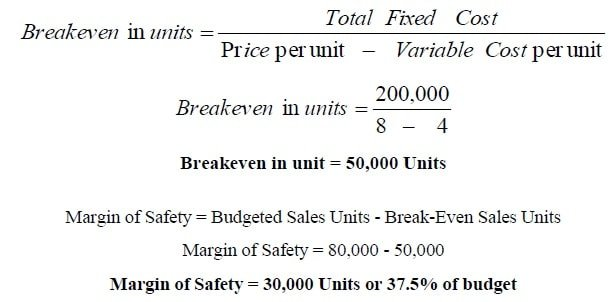>> Practice Cost Volume Profit Analysis Quiz 1 and Quiz 2.